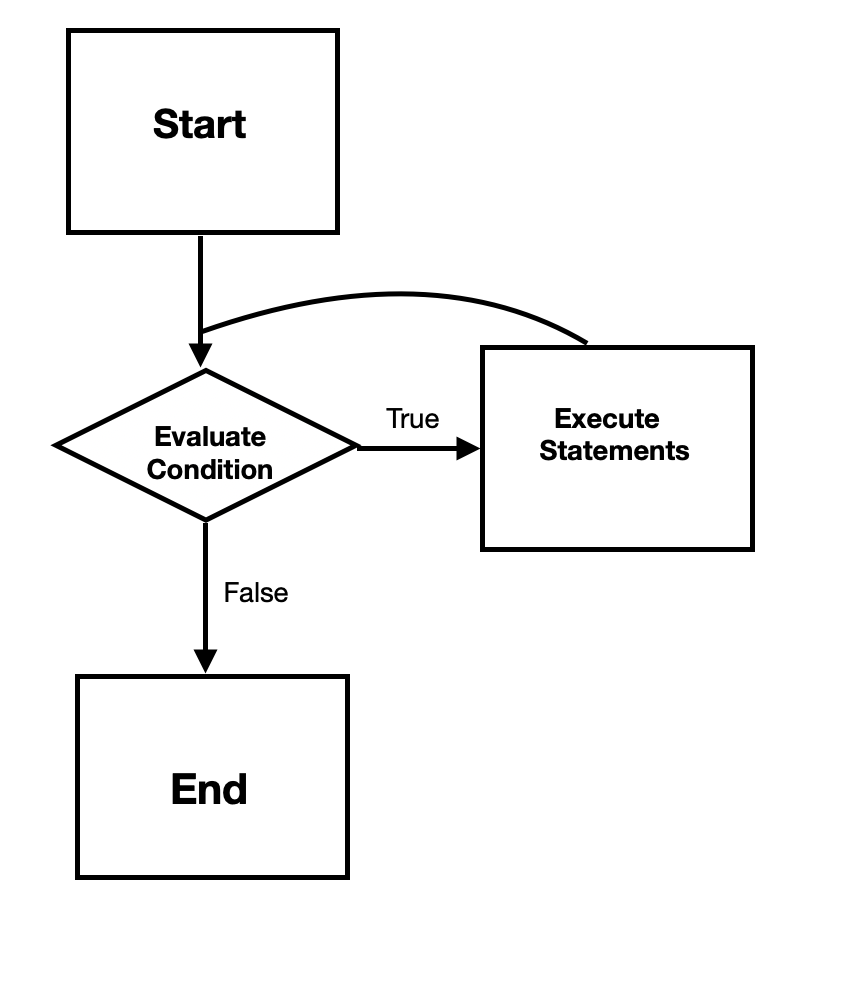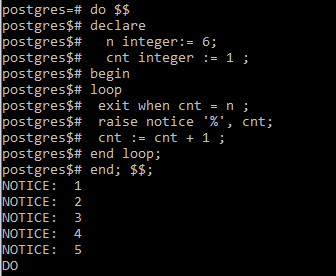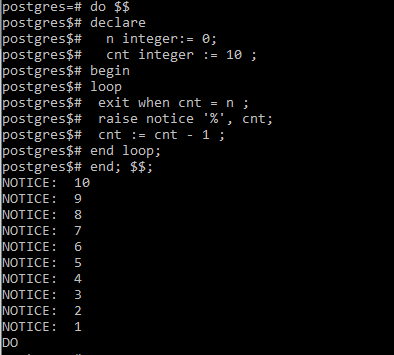# PostgreSQL – Loop Statement

The loop statement is used to simply define an unconditional loop that executes statements of code repeatedly until it is terminated after encountering a return or exit statement.

Syntax:

```<<label>>
loop
statements/body;
end loop;```

In the above syntax, we must ideally do the following :

• Condition for termination:  We should try to define a condition in the body of the loop until which our loop runs. As soon as this condition fails, the loop will be terminated. If we do not define a termination condition, the loop executes infinite times and we encounter the case of an infinite loop.

• Increment/Decrement: We should try to include an increment or decrement statement inside the body to increase or decrease the running variable. If we do not change the value of the variable, the loop gets stuck in the same condition again and again and we encounter the case of an infinite loop.

To terminate the running of the loop, we can simply include an if statement with an exit statement with the following syntax:

```<<label>>
loop
statements;
if condition then
exit;
end if;
end loop;```A condition when we place a loop inside another loop is called a nested loop. It is important to note that whenever we use nested looping, we must define the loop labels in the exit or continue statements to show precisely which loop we are referring to.

```<<outer>>
loop
statements;
<<inner>>
loop
inside statements;
exit <<inner>>
end loop;
end loop;```

Example 1:

The following example shows how to use the loop statement to print all numbers from 1 to 5.

```do \$\$
declare
n integer:= 6;
cnt integer := 1 ;
begin
loop
exit when cnt = n ;
raise notice '%', cnt;
cnt := cnt + 1 ;
end loop;
end; \$\$;```

Output:In the above example, we define a cnt variable whose value is increased at each iteration. The value of cnt is printed until it reaches our peak value of n after which the loop is terminated.

Example 2:

The following example shows how to use the loop statement to print all numbers from 10 to 1.

```do \$\$
declare
n integer:= 0;
cnt integer := 10 ;
begin
loop
exit when cnt = n ;
raise notice '%', cnt;
cnt := cnt - 1 ;
end loop;
end; \$\$;```

Output:In the above example, we define a cnt variable whose value is decreased at each iteration. The value of cnt is printed until it reaches our minimum value of n after which the loop is terminated.

Whether you're preparing for your first job interview or aiming to upskill in this ever-evolving tech landscape, GeeksforGeeks Courses are your key to success. We provide top-quality content at affordable prices, all geared towards accelerating your growth in a time-bound manner. Join the millions we've already empowered, and we're here to do the same for you. Don't miss out - check it out now!

Previous
Next